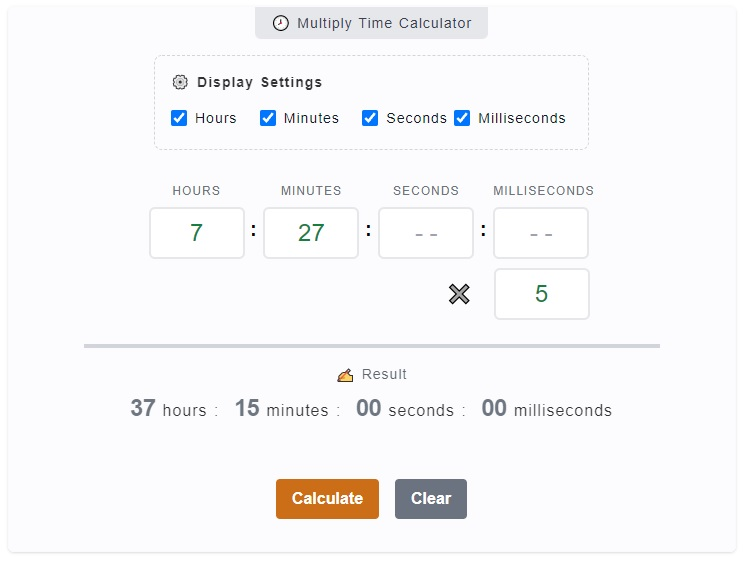# Multiply Time Calculator

The Multiply Time Calculator is used to get the product of time multiplied by a whole number. In other words, the purpose of this calculator is to answer questions like this, what is `7 hours and 27 minutes` multiplied by `7`? You can enter these values on the calculator and you'll get the result.

🕗 Multiply Time Calculator

:
:
:
✖️

### ✍ Result

00 hours : 00 minutes
00 seconds : 00 milliseconds

## How to Use the Multiply Time Calculator

This calculator is quite useful when you are dealing with multiplying complex times. What it means is that, when you multiply 2 hours by 4, it's easy to know that the answer is 8 hours. However, when you are to multiply 13 hours: 17 minutes by 13, this is rather challenging for some. This is where this calculator comes to the rescue.1. Step 1

The first thing you might want to do is to check whether you need all hours, minutes, seconds and milliseconds input fields. You can omit the one's you don't need by just unchecking the corresponding checkbox on the `⚙️ Display Settings`.

2. Step 2

Then, we proceed to the actual entering of time. On the calculator, there are input fields marked with hours, minutes, seconds and milliseconds. Enter your base time you want to multiply on these fields. In mathematical multiplication, it's called multiplicand. For example, enter 13 hours, 14 minutes, 30 seconds and 50 ms.

3. Step 3

Following our example time entry above (`13 hours, 14 minutes, 30 seconds and 50 ms`). We can now enter the multiplier which is the input field beside the multiply (✖️) sign. It is the number we want to multiply our time entry with. Let's add `7` on this field.

4. Step 4

After entering both the time (multiplicand) and (multiplier), you can now click on the `Calculate` button to initialize the computation. The result will be displayed at the bottom part of the calculator labeled with `✍ Result.`

## Multiply Time Calculator Inputs and Outputs

The purpose of the calculator is to give you the result when you multiply a time (HH:MM:SS) by a whole number. You can just check around the calculator and you'll be able to use it correctly. However, mentioning the inputs and outputs of this calculator would provide a better information.

### Display Settings

The display settings are added on the calculator for you to personalize your inputs. These are in the form of checkboxes, which is checked by default. It includes the `Hours`, `Minutes`, `Seconds` and `Milliseconds`. You can actually uncheck the one's you don't need and it will be hidden on the display.

### Inputs

There are two (input) groups on the calculator. The first one is the multiplicand which represents your time entry. Then, the other one is the multiplier which is a number used to multiply your time with.

### Outputs

The output or the result of the calculation is being displayed right below the input fields which is labeled with `✍ Result`. This serves as the output or product when multiplying time and a number.

### Calculate and Clear Buttons

Make sure to click on the `Calculate` button every time you have finished entering your inputs. You can also clear them by just clicking the `Clear` button.

## Video Explanation

Here's a video tutorial on how to use the Multiply Time Calculator.